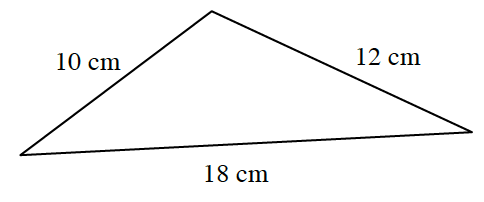### Home > PC > Chapter 2 > Lesson 2.3.6 > Problem2-145

2-145.Find the area of the triangle to the right.

Use the Law of Cosines to determine the measure of one of the angles.

Use the formula below to calculate the area.

$A=\frac{1}{2}ab\sin(\theta)$